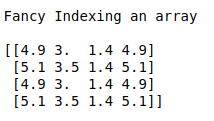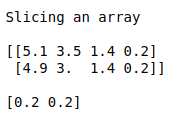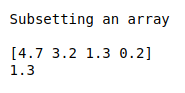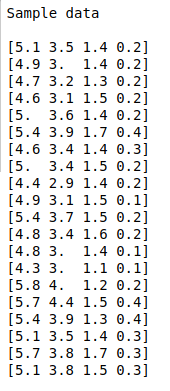• #5, First Floor, 4th Street Dr. Subbarayan Nagar Kodambakkam, Chennai-600 024 Landmark : Samiyar Madam
• pro@slogix.in
• +91- 81240 01111

### How to do Boolean indexing, Fancy indexing and sub setting of an array using Numpy?

###### Description

To write a piece of python code for Sub setting and indexing of Numpy array.

Numpy array.

#### Output

Sub set of an array

Boolean indexing of an array

Fancy indexing of an array

###### Process

Create an array.

Do indexing and slicing of an array using appropriate procedures.

Print the results.

###### Sample Code

#import libraries
import numpy as np
import pandas as pd

#load the sample data from csv file

#Make it as a data frame
df = pd.DataFrame(data)

#feature selection
X = np.array(df.iloc[:,0:4])
print(“Sample data\n”)
for i in range(20):
print(X[i])
print(“\n”)

#Subsetting of an array
print(“Subsetting an array\n”)
print(X)
print(X[2,2])
print(“\n”)

#Slicing of an array
print(“Slicing an array\n”)
print(X[0:2],”\n”)
print(X[0:2,3])
print(“\n”)

#Boolean indexing of an array
print(“Boolean indexing\n”)
print(X[X<3])
print(“\n”)

#Fancy indexing of an array
print(“Fancy Indexing an array\n”)
print(X[[1, 0, 1, 0]][:,[0,1,2,0]])

###### Screenshots